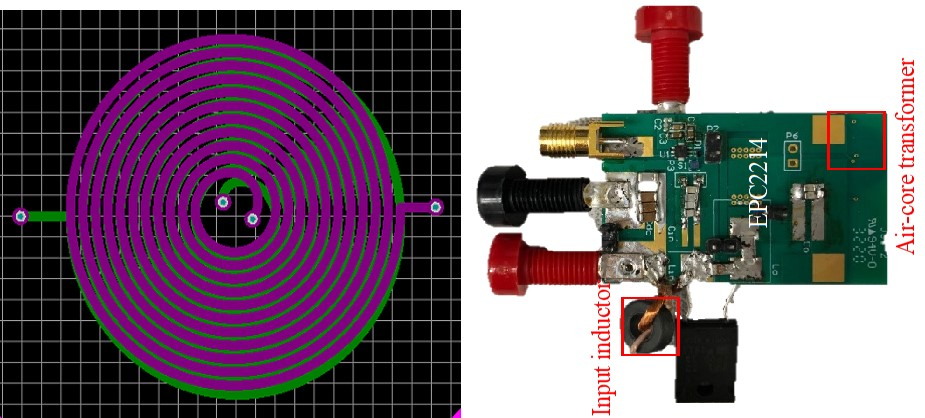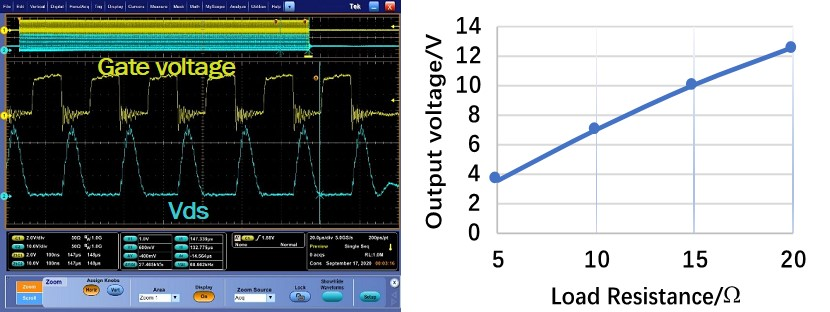LIBRARY

# A Class-E Gate Power Supply with Air-Core TransformerFig. 1. The drawing of the air-core transformer and the hardware.
Current source is used in gate drivers, battery chargers and wireless power transfer. Class-E converter is a good candidate for the application because only one switch is required and ZVS is achieved. Class-E inverter with constant-current (CC) output and ZVS over a wide load range with finite input inductance has been proposed. An isolation stage needs to be added to fit with the requirement of gate driver and battery charger. An air-core transformer is used for lower profile and to eliminate core loss. The air-core transformer with PCB winding has two planar layers. The radius is 5 mm. The self-inductance is 700 nH and mutual-inductace is 360 nH. The drawing of the air core transformer and the hardware are shown in Fig. 1.

The class-e inverter can be equivalized with a voltage source in series with a capacitor. The fundamental output voltage can be constant if the capacitor is compensated at certain switching frequency and the load is resistive. The CC output is realized by adding a CLC network to the class-e inverter. Capacitor C1 and C2 compensate the self-inductance at the primary side and secondary side to realize a resistive load. For duty ratio (D) equal to 0.5, the switching frequency needs to be 1.29 of the resonant frequency of Lin and Cin. The leakage inductaces Ll1 and Ll2 need to be large enough to suppress high-order harmonics. In this work, the self-inductance is 600 nH and mutual-inductace is 360 nH. The switching frequency is 6.78 MHz and the output current is 700 mA.

Fig.2. shows the testing results with 10 V input and different load-resistances. ZVS is always maintained within the load range and output voltage increases linearly with the load-resistance, which is the characteristics of CC output. The peak efficiency of the converter is 80%.

In summary, this work demonstrated the principle of realizing CC output with class-e converter and an air-core transformer. The work can be a good candidate for high-temperature and low-profile applications with the elimination of magnetic cores. The efficiency can be further improved by optimizing the air-core transformer and will be discussed in the future.Fig. 2.Testing waveforms and outptut characteristic of the converter
,